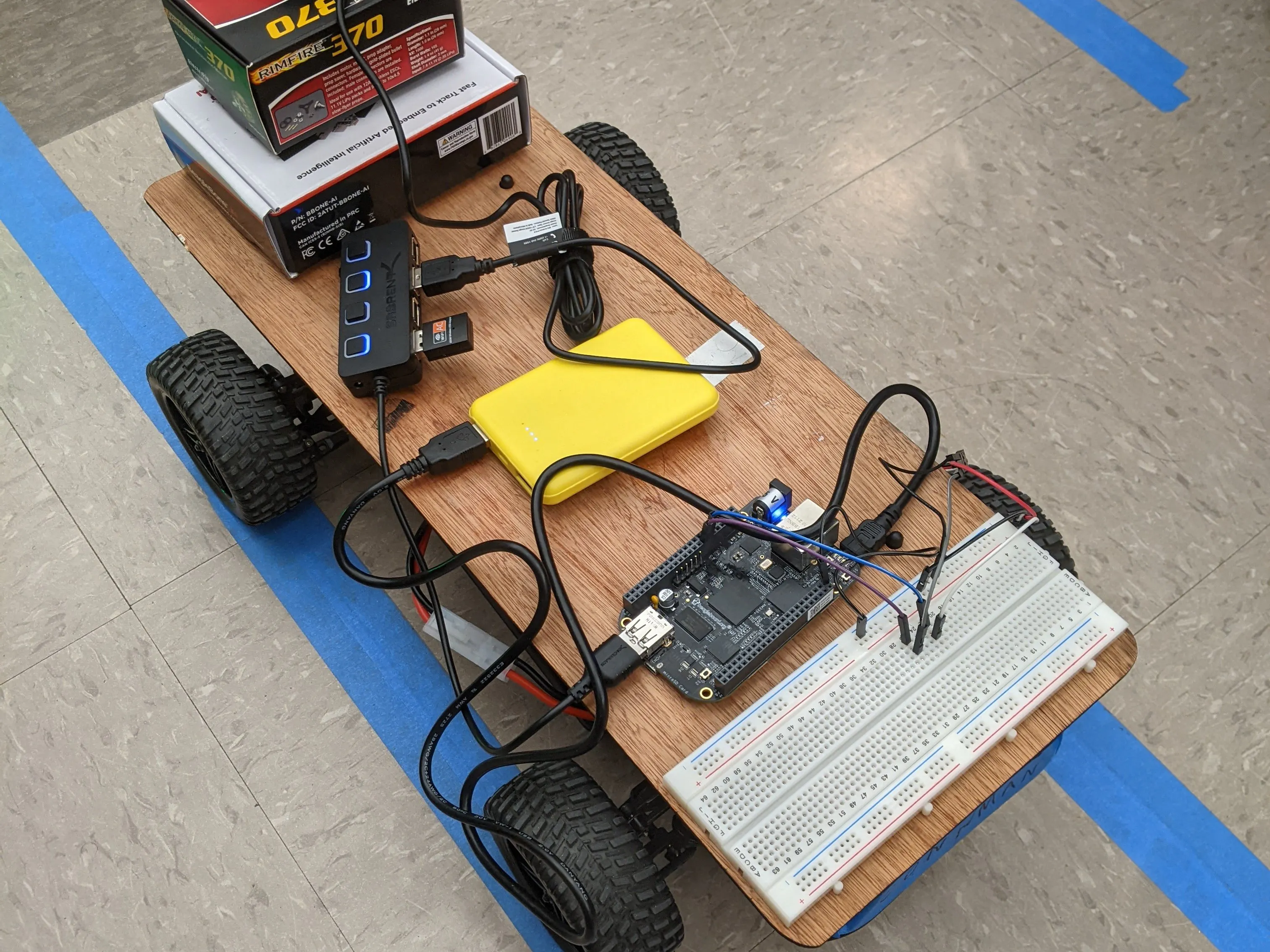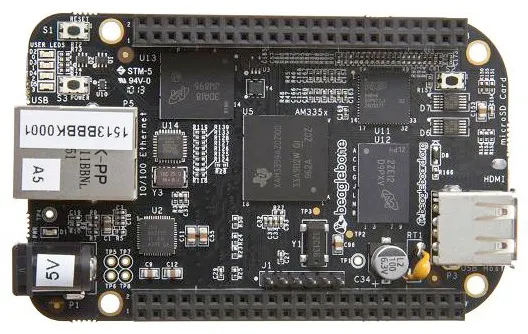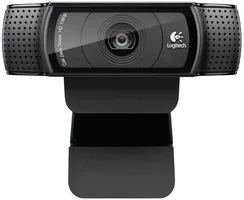BeagleCar

Designing a car to stay within lane and react to stop signs and lights

IntermediateShowcase (no instructions)20 hours6Things used in this project

Hardware componentsBeagleBoard.org BeagleBone Black It survived.
×1Webcam, Logitech® HD Pro
×1
×1
 USB Hub
×1

Software apps and online servicesOpenCV

Code

rc.py

Python
# The following code is modified from User raja_961
# "Autonomous Lane-Keeping Car Using Raspberry Pi and OpenCV".
# Instructables.
# URL: https://www.instructables.com/Autonomous-Lane-Keeping-Car-Using-Raspberry-Pi-and/
import cv2
import functools
import numpy as np
import math
import sys
import time

#########################################################
############### Pin connections for motors ##############
#########################################################

ESC = "P9_16"
SERVO = "P9_14"

#########################################################
##### Tunable values based on car and environment #######
#########################################################

# Left-most and right-most PWM turning values
MIN_TURN = 6.5
MAX_TURN = 8.5

# Min and max angle deviations from center
MIN_DEVIATION = -10
MAX_DEVIATION = 10

# Resize frame for faster processing
FRAME_WIDTH = 60
FRAME_HEIGHT = 45

# Amount of stop sign red to detect to stop
STOP_SIGN_THRESHOLD = 0.1

# Speed bump after stopping to overcome static friction
SPEED_INCREASE_AFTER_STOP = 0.08
SPEED_DECREASE_AFTER_STOP = 0.06

# Amount of stop light color to detect
STOP_LIGHT_RED_THRESHOLD = 0.001
STOP_LIGHT_GREEN_THRESHOLD = 0.001

#########################################################
################ Lane tracking functions ################
#########################################################

def detect_edges(frame):
# filter for blue lane lines
hsv = cv2.cvtColor(frame, cv2.COLOR_BGR2HSV)
# cv2.imshow("HSV",hsv)
lower_blue = np.array([90, 120, 0], dtype="uint8")
upper_blue = np.array([150, 255, 255], dtype="uint8")

# detect edges
# cv2.imshow("edges",edges)
return edges

def region_of_interest(edges):
height, width = edges.shape
# only focus lower half of the screen
polygon = np.array([[
(0, height),
(0,  height/2),
(width, height/2),
(width, height),
]], np.int32)
# cv2.imshow("roi",cropped_edges)
return cropped_edges

def detect_line_segments(cropped_edges):
rho = 1
theta = np.pi / 180
min_threshold = 10

line_segments = cv2.HoughLinesP(cropped_edges, rho, theta, min_threshold,
np.array([]), minLineLength=5, maxLineGap=150)
return line_segments

def average_slope_intercept(frame, line_segments, debug=True):
lane_lines = []
if line_segments is None:
if debug:
print("no line segments detected")
return lane_lines

height, width, _ = frame.shape
left_fit = []
right_fit = []

boundary = 1/3
left_region_boundary = width * (1 - boundary)
right_region_boundary = width * boundary

for line_segment in line_segments:
for x1, y1, x2, y2 in line_segment:
if x1 == x2:
if debug:
print("skipping vertical lines (slope = infinity")
continue
fit = np.polyfit((x1, x2), (y1, y2), 1)
slope = (y2 - y1) / (x2 - x1)
intercept = y1 - (slope * x1)
if slope < 0:
if x1 < left_region_boundary and x2 < left_region_boundary:
left_fit.append((slope, intercept))
else:
if x1 > right_region_boundary and x2 > right_region_boundary:
right_fit.append((slope, intercept))

left_fit_average = np.average(left_fit, axis=0)
if len(left_fit) > 0:
lane_lines.append(make_points(frame, left_fit_average))

right_fit_average = np.average(right_fit, axis=0)
if len(right_fit) > 0:
lane_lines.append(make_points(frame, right_fit_average))

return lane_lines

def make_points(frame, line):
height, width, _ = frame.shape
slope, intercept = line
y1 = height  # bottom of the frame
y2 = int(y1 / 2)  # make points from middle of the frame down
if slope == 0:
slope = 0.1
x1 = int((y1 - intercept) / slope)
x2 = int((y2 - intercept) / slope)
return [[x1, y1, x2, y2]]

def display_lines(frame, lines, line_color=(0, 255, 0), line_width=6):
line_image = np.zeros_like(frame)
if lines is not None:
for line in lines:
for x1, y1, x2, y2 in line:
cv2.line(line_image, (x1, y1), (x2, y2),
line_color, line_width)
line_image = cv2.addWeighted(frame, 0.8, line_image, 1, 1)
return line_image

def display_heading_line(frame, steering_angle, line_color=(0, 0, 255), line_width=5):
height, width, _ = frame.shape
steering_angle_radian = steering_angle / 180.0 * math.pi
x1 = int(width / 2)
y1 = height
x2 = int(x1 - height / 2 / math.tan(steering_angle_radian))
y2 = int(height / 2)

cv2.line(heading_image, (x1, y1), (x2, y2), line_color, line_width)

def get_steering_angle(frame, lane_lines):
height, width, _ = frame.shape
if len(lane_lines) == 2:
_, _, left_x2, _ = lane_lines
_, _, right_x2, _ = lane_lines
mid = int(width / 2)
x_offset = (left_x2 + right_x2) / 2 - mid
y_offset = int(height / 2)
elif len(lane_lines) == 1:
x1, _, x2, _ = lane_lines
x_offset = x2 - x1
y_offset = int(height / 2)
elif len(lane_lines) == 0:
x_offset = 0
y_offset = int(height / 2)
angle_to_mid_deg = int(angle_to_mid_radian * 180.0 / math.pi)
steering_angle = angle_to_mid_deg + 90
return steering_angle

def detect_color(low_range, high_range, frame, imshow=False):
"""
Returns a mask of a frame for the color of a given range.
"""
hsv = cv2.cvtColor(frame, cv2.COLOR_BGR2HSV)
if imshow:

"""
Returns True if the given mask passes the threshold.
"""
return count / mask.size > threshold

#########################################################
################## Stop sign functions ##################
#########################################################

def detect_red_stop_sign(frame, imshow=False):
return detect_color((170, 50, 20), (185, 255, 255), frame, imshow=imshow)

#########################################################
################# Stop light functions ##################
#########################################################

def detect_red_stop_light(frame, imshow=False):
return detect_color((0, 70, 250), (10, 120, 255), frame, imshow=imshow)

def detect_green_stop_light(frame, imshow=False):
return detect_color((60, 90, 230), (85, 120, 255), frame, imshow=imshow)

#########################################################
############## Car manipulation functions ###############
#########################################################

def reset_all():
"""
Reset all motors.
"""
PWM.set_duty_cycle(SERVO, 7.5)
PWM.set_duty_cycle(ESC, 7.5)

def turn(value):
"""
Turn the vehicle by the value.
"""
PWM.set_duty_cycle(SERVO, value)

def set_speed(speed):
"""
Set the speed for the vehicle.
"""
PWM.set_duty_cycle(ESC, speed)

def compute_steering_deviation(frame, imshow=False):
"""
Given a video frame, detect the deviation from the center.
"""
edges = detect_edges(frame)
roi = region_of_interest(edges)
line_segments = detect_line_segments(roi)
lane_lines = average_slope_intercept(frame, line_segments)
lane_lines_image = display_lines(frame, lane_lines)
steering_angle = get_steering_angle(frame, lane_lines)
if imshow:
return steering_angle - 90

def turn_after_deviation(deviation):
"""
Given a deviation value, turn to steer towards the center.
"""
# Linearly interpolate steering if within range.
if MIN_DEVIATION < deviation and deviation < MAX_DEVIATION:
turn_value = (MAX_TURN - MIN_TURN) / \
(MAX_DEVIATION - MIN_DEVIATION) * deviation + 7.5
turn(turn_value)
return turn_value
elif deviation > MAX_DEVIATION:
# Vehicle is turned too far, but don't turn back too much (overcorrect).
turn(MIN_TURN)  # Clamp left turn
return MIN_TURN
elif deviation < MIN_DEVIATION:
turn(MAX_TURN)  # Clamp right turn
return MAX_TURN

def handle_exception(func):
"""
Python decorator to automatically reset motors when
SIGINT (Ctrl + C) is received.
"""
@functools.wraps(func)
def func_wrapper(*args, **kwargs):
try:
return func(*args, **kwargs)
except KeyboardInterrupt:
print("\nRecalibrating...\n")
reset_all()
return None
return func_wrapper

#########################################################
################### Top level functions #################
#########################################################

# @handle_exception
def run(speed=7.5, should_turn=True, cam=False, stop=False, stopcam=False, stoplight=False):
# Optimization: Since a single stoplight occurs before the two stop signs,
# we check for a stop light first (and not for any stop sign).
# After the stop light is passed, we stop checking for stop lights and start
# checking for stop signs.
passed_stoplight = not stoplight
has_just_stopped = False
n = 0  # Frame number
vals = [('error', 'turning_pwm', 'speed_pwm')]  # Collect values for graph
try:
while True:
# Get video and resize frame.
frame = cv2.resize(frame, (FRAME_WIDTH, FRAME_HEIGHT))

# Detect lanes and steer towards the center.
deviation = compute_steering_deviation(frame, imshow=cam)
error = abs(deviation)
set_speed(speed)
if should_turn:
t = turn_after_deviation(deviation)
turn_value = t if t else turn_value

if cam:
cv2.waitKey(1)

# Check for stoplights.
if stoplight and not passed_stoplight:
set_speed(7.5)  # Stop!
vals.append((error, turn_value, speed))
# Found red stop light. Wait until light turns green.
while True:
# Check video frame again until light turns green.
frame = cv2.resize(frame, (FRAME_WIDTH, FRAME_HEIGHT))
passed_stoplight = True
set_speed(speed)  # Green light. Go!
break

# Check for stop signs every 3 frames.
if stop and n % 3 == 0 and passed_stoplight:
# Just found a stop sign!
if not has_just_stopped and is_at_stop_sign:
set_speed(7.5)
turn(7.5)
time.sleep(3)  # Wait for 3 seconds.
has_just_stopped = True
speed += SPEED_INCREASE_AFTER_STOP
set_speed(speed)
elif has_just_stopped and not is_at_stop_sign:
# Just passed stop sign, we can slow down after the speed increase now.
has_just_stopped = False
speed -= SPEED_DECREASE_AFTER_STOP
if stopcam:
cv2.waitKey(1)
vals.append((error, turn_value, speed))
n += 1
except KeyboardInterrupt:
reset_all()
with open('errors.csv', 'w') as f:
f.write(','.join(vals) + '\n')
for item in vals[1:]:
f.write("%f,%f,%f\n" % item)

def debug_color(detect_func, cam=False, print_percent=False):
"""
Detect a color for debugging.
-- cam shows the mask for the color.
-- print_percent prints the percent of the color in the mask.
"""
while True:
if cam:
cv2.waitKey(1)
if print_percent:

@handle_exception
def debug_stop_sign(cam=False, print_percent=False):
debug_color(detect_red_stop_sign, cam=cam, print_percent=print_percent)

@handle_exception
def debug_red_stop_light(cam=False, print_percent=False):
debug_color(detect_red_stop_light, cam=cam, print_percent=print_percent)

@handle_exception
def debug_green_stop_light(cam=False, print_percent=False):
debug_color(detect_green_stop_light, cam=cam, print_percent=print_percent)

@handle_exception
def debug_stop_light2(cam=False, print_percent=False, line_color=(0, 0, 255), line_width=5):
while True:
height, width, _ = frame.shape
line_image = np.zeros_like(frame)
cv2.line(line_image, (0, height//2),
(width//2, height//2), line_color, line_width)
cv2.line(line_image, (width//2, 0),
(width//2, height//2), line_color, line_width)
line_image = cv2.addWeighted(frame, 0.8, line_image, 1, 1)
hsv = cv2.cvtColor(frame, cv2.COLOR_BGR2HSV)
height, width, _ = hsv.shape
print(hsv[height//2, width//2])
cv2.imshow("frame", line_image)
cv2.waitKey(1)

def stop():
video.release()
cv2.destroyAllWindows()
PWM.stop(ESC)
PWM.stop(SERVO)

#########################################################
############### Initialize video and motors #############
#########################################################

video = cv2.VideoCapture(0)
video.set(cv2.CAP_PROP_FRAME_WIDTH, 320)
video.set(cv2.CAP_PROP_FRAME_HEIGHT, 240)

PWM.start(ESC, 7.5, 50, 0)
PWM.start(SERVO, 7.5, 50, 0)

Credits

Shaquille Que

1 project • 0 followers

Andrew Obler

1 project • 0 followers

Daniel Puckett

1 project • 0 followers
Thanks to raja_961.